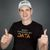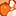# QlikView Documents

QlikView documentation and resources.

Announcements
Document boards are being consolidated, this board no longer allows NEW documents READ MORE

# Convert Gauss-Kruger (GK) coordinates to Latitude/Longitude

cancel
Showing results for
Did you mean:Partner - Creator II

## Convert Gauss-Kruger (GK) coordinates to Latitude/Longitude

Background:

In cartography, the term Gauss–Krüger Geographic coordinate system is named after Carl Friedrich Gauss (1777-1855) and Johann Heinrich Louis Krüger. It is a particular set of transverse Mercator projections (map projections) used in narrow zones in Europe and South America, at least in Germany, Turkey, Austria, Slovenia, Croatia, Macedonia, Finland and Argentina. This Gauss–Krüger system is similar to the universal transverse Mercator system (UTM), but the central meridians of the Gauss–Krüger zones are only 3° apart, as opposed to 6° in UTM. Depending on the zone of the Gauss-Kruger coordinates, different EPSG codes are applicable (The country of Germany is within the EPSG-Codes 31466 to 31469). Nevermind about the EPSG code, the below conversion works on any Gauss-Kruger coordinates.

The typical Gauss-Krüger coordinates is a pair of "Rechtswert" (East) and "Hochwert" (North) coordinates, both numbers are 7 digits long, may have up to 3 decimals (since the unit is meter, decimals don't make much sense as they are about decimeters, centimeters, even millimeter).

Solution:

Enough about the background, to convert given set of Gauss-Krüger coordinates from a given "Rechtswert" (East) and "Hochwert" (North) in QlikView add the following script fragments. Adjust the values of XCOORD_Field and Y_COORD Field with the effective column names in which the GK values are found in the later LOAD statement.

// constants

LET XCOORD_Field = 'XKOORD';

LET YCOORD_Field = 'YKOORD';

// Formulas
SET @rho = 57.29577951; //=180/PI

SET @e2 = 0.006719219;

SET @b1 = "(\$(YCOORD_Field)/10000855.7646)";

SET @b2 = "Pow(\$(@b1),2)";

SET @bf = "325632.08677*\$(@b1)*((((((0.00000562025*\$(@b2)-0.0000436398)*\$(@b2)+0.00022976983)*\$(@b2)-0.00113566119)

*\$(@b2)+0.00424914906)*\$(@b2)-0.00831729565)*\$(@b2)+1) / 3600/ \$(@rho)";

SET @fa = "((\$(XCOORD_Field)-(Floor(\$(XCOORD_Field)/1000000)*1000000)-500000)/(6398786.849/Sqrt(1+(Pow(COS(\$(@bf)),2)*\$(@e2)))))";

SET @LAT_Formula = "(\$(@bf)-(Pow(\$(@fa),2)*(Sin(\$(@bf))/Cos(\$(@bf)))*(1+(Pow(COS(\$(@bf)),2) * \$(@e2)))/2)

+(Pow(\$(@fa),4)*(Sin(\$(@bf))/Cos(\$(@bf)))*(5+(3*Pow(Sin(\$(@bf))/Cos(\$(@bf)),2))+(6*(Pow(COS(\$(@bf)),2)

* \$(@e2)))-(6*(Pow(COS(\$(@bf)),2) * \$(@e2))*Pow(Sin(\$(@bf))/Cos(\$(@bf)),2)))/24)) * \$(@rho)";

SET @LON_Formula = "((\$(@fa)-(Pow(\$(@fa),3)*(1+(2*Pow(Sin(\$(@bf))/Cos(\$(@bf)),2))+(Pow(COS(\$(@bf)),2)

* \$(@e2)))/6)+(Pow(\$(@fa),5)*(1+(28*Pow(Sin(\$(@bf))/Cos(\$(@bf)),2))+(24*Pow(Sin(\$(@bf))/Cos(\$(@bf)),4)))/120))

* \$(@rho)/COS(\$(@bf))) + (Floor(\$(XCOORD_Field)/1000000)*3)";

Now if you import a file or table with Gauss-Krüger coordinates in fields XKOORD / YKOORD this is your script (dark-blue part). If you plan to use QlikView's built-in Mapping Extension "Quick Map" or "QlikView Mapping Extension", the coordinates-pair needs to go into one field, which I am calling LON_LAT. They need to have US number format. use the dark-red part of the script as well.

SET US_Format = "'','.',' '";

*

,Num(LON,\$(US_Format)) & ',' & Num(LAT,\$(US_Format)) AS LON_LAT

;

ID

,XKOORD,

,YKOORD

,\$(@LAT_Formula) AS LAT

,\$(@LON_Formula) AS LON

FROM

[myExcel.xlsx]

(ooxml, embedded labels);

Enjoy,

Christof

Tags (4)
Labels (3)

• ### ScriptingNot applicable

Great work!

I am trying to utilize this in Finland with GK25. It seems to, however, give incorrect coordinates. You mentioned in your text that the formula should work in any projection strip if I understood correctly.

Is there some parameters that I should adjust to make it work? It would be stunning to be able to utilize this instead of additional conversion tasks from the database.

BR,

ErnoPartner - Creator II

can you send me the Qlikview file with the non working Geo points? Csw@qlik.com<mailto:Csw@qlik.com>

Von meinem iPhone gesendet

Am 26.04.2016 um 18:18 schrieb Erno Bister <qcwebmaster@qlikview.com<mailto:qcwebmaster@qlikview.com>>:Contributor

Awesome work with the script! I have tried to incorporate it in a Qlik Sense application, but have some difficulties with the longitude-field as I get totally wrong results.

I am located in Sweden and using coordinates from RT90 2.5 gon V EPSG: 3021.  I havn't made any alterations to your script, but has the following input for the constants:

``````LET XCOORD_Field = 1629750;
LET YCOORD_Field = 6580600;``````

Which give me the following result:

LAT: 59,326740142441

LON: 5,2796907841121

LAT is close to desired location but LON i far off. Right coordinates should be something like:

LAT: 59,32626

LON: 18,08452

Do I maybe have to do some alterations in the script based on the swedish projection I am using? I found this PDF with Krüger's formulas and the constants for the actual projection, but having difficulties seeing what changes can be made directly.

Any help will be highly appreciated!Contributor

Given the geodetic coordinate points with GRS80 ellipsoid as datum, how can i  calculate the corresponding Gauss-Kruger 6 degree zone plane coordinates.Contributor

Great work with the script.

I'm trying to convert from zone 7N to wgs84, but i'm getting wrong coordinates.

Is there same constants that needs to be changed?

Version history
Last update:
‎2014-06-30 11:16 AM
Updated by: